# piecewiseSEM: Piecewise Structural Equation Modeling in R

#### 2020-12-09

Structural equation modeling (SEM) is among the fastest growing statistical techniques in ecology and evolution, and provides a new way to explore and quantify ecological systems. SEM unites multiple variables in a single causal network, thereby allowing simultaneous tests of multiple hypotheses. The idea of causality is central to SEM as the technique implicitly assumes that the relationships among variables represent causal links. Because variables can be both predictors and responses, SEM is also a useful tool for quantifying both direct and indirect (cascading) effects.

Piecewise SEM (or confirmatory path analysis) expands upon traditional SEM by introducing a flexible mathematical framework that can incorporate a wide variety of model structures, distributions, and assumptions. These include: interactions and non-normal responses, random effects and hierarchical models, and alternate correlation structures (including phylogenetic, spatial, and temporal).

This release is version 2.0 of the package and contains substantial updates to both the syntax and the underlying calculations. All functions have been replaced and rewritten from the ground up.

The first part of this vignette will briefly introduce the concepts behind piecewise SEM. The second part will introduce the new syntax using a worked example. The final part will briefly compare the old and new versions of the package.

## 1. An Introduction to Structural Equation Modeling

Broadly, structural equation modeling (SEM) unites a suite of variables in a single network. They are generally presented using box-and-arrow diagrams denoting directed (causal) relationships among variables: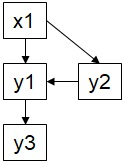1.1 Example SEM

Those variables that exist only as predictors in the network are referred to as exogenous, and those that are predicted (at any point) as endogenous. Exogenous variables therefore only ever have arrows coming out of them, while endogenous arrows have arrows coming into them (which does not preclude them from having arrows come out of them as well). This vocabulary is important when considering some special cases later.

In traditional SEM, the relationships among variables (i.e., their linear coefficients) are estimated simultaneously in a single variance-covariance matrix. This approach is well developed but can be computationally intensive (depending on the sizes of the v-cov matrix) and additionally assumes independence and normality of errors, two assumptions that are generally violated in ecological research.

Piecewise structural equation modeling (SEM), also called confirmatory path analysis, was proposed in the early 2000s by Bill Shipley as an alternate approach to traditional variance-covariance based SEM. In piecewise SEM, each set of relationships is estimated independently (or locally). This process decomposes the network into the corresponding simple or multiple linear regressions for each response, each of which are evaluated separately, and then combined later to generate inferences about the entire SEM. This approach has two consequences: 1. Increasingly large networks can be estimated with ease compared to a single vcov matrix (because the approach is modularized), and 2. Specific assumptions about the distribution and covariance of the responses can be addressed using typical extensions of linear regression, such as fixed covariance structures, random effects, and other sophisticated modeling techniques.

Unlike traditional SEM, which uses a $$\chi^2$$ test to compare the observed and predicted covariance matrices, the goodness-of-fit of a piecewise structural equation model is obtained using ‘tests of directed separation.’ These tests evaluate the assumption that the specific causal structure reflects the data. This is accomplished by deriving the ‘basis set,’ which is the smallest set of independence claims obtained from the SEM. These claims are relationships that are unspecified in the model, in other words paths that could have been included but were omitted because they were deemed to be biologically or mechanistically insignificant. The tests ask whether these relationships can truly be considered independent (i.e., their association is not statistically significant within some threshold of acceptable error, typically $$\alpha$$=0.05) or whether some causal relationship may exist as indicated by the data.

For instance, the preceding example SEM contains 4 specified paths (solid, black) and 2 unspecified paths (dashed, red), the latter of which constitute the basis set: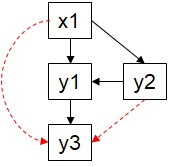1.2 Missing Paths

In this case, there are two relationships that need to be evaluated: y3 and x1, and y3 and y2. However, there are additional influences on y3, specifically the directed path from y2. Thus, the claims need to be evaluated for ‘conditional independence,’ i.e. that the two variables are independent conditional on the already specified influences on both of them. This also pertains to the predictors of y2, including the potential contributions of x1. So the full claim would be: y2 | y3 (y1, x1), with the claim of interest separated by the | bar and the conditioning variable(s) following in parentheses.

As the network grows more complex, however, the independence claims only consider variables that are immediately ancestral to the primary claim (i.e., the parent nodes). For example, if there was another variable predicting x1, it would not be considered in the independence claim between y3 and y2 since it is >1 node away in the network.

The independence claims are evaluated by fitting a regression between the two variables of interest with any conditioning variables included as covariates. Thus, the claim above y2 | y3 (y1, x1) would be modeled as y3 ~ y2 + y1 + x1 . These regressions are constructed using the same assumptions about y3 as specified in the actual structural equation model. So, for instance, if y3 is a hierarchically sampled variable predicted by y1, then same hierarchical structure would carry over to the test of directed separation of y3 predicted by y2.

The P-values of the conditional independence tests are then combined in a single Fisher’s C statistic using the following equation:

$C = -2\sum_{i=1}^{k}ln(p_{i})$

This statistic is $$\chi^2$$-distributed with 2k degrees of freedom, with k being the number of independence claims in the basis set.

Shipley (2013) also showed that the the C statistic can be used to compute an AIC score for the SEM, so that nested comparisons can be made in a model selection framework:

$AIC = C + 2K$

where K is the likelihood degrees of freedom. A further variant, $$AIC_c$$, can be obtained by adding an additional penalty based on sample size:

$AIC_c = C + 2K\frac{n}{(n - K - 1)}$

The piecewiseSEM package automates the derivation of the basis set and the tests of directed separation, as well as extraction of path coefficients based on the user-specified input.

## 2. An Example using piecewiseSEM

### 2.1 Worked example

Let’s make up some fake data corresponding to the path diagram above:

dat <- data.frame(x1 = runif(50), y1 = runif(50), y2 = runif(50), y3 = runif(50))

And we will use piecewiseSEM to fit the model. The primary function is psem and we supply the regressions corresponding to the relationships specified in the path diagram, separated by commas as in a list:

# Load required libraries
library(piecewiseSEM)
## Registered S3 methods overwritten by 'lme4':
##   method                          from
##   cooks.distance.influence.merMod car
##   influence.merMod                car
##   dfbeta.influence.merMod         car
##   dfbetas.influence.merMod        car
##
##   This is piecewiseSEM version 2.1.0.
##
##
##   Questions or bugs can be addressed to <[email protected]>.
model <- psem(lm(y1 ~ x1, dat), lm(y1 ~ y2, dat), lm(y2 ~ x1, dat), lm(y3 ~ y1, dat))
## Error: Duplicate responses detected in the model list. Collapse into single multiple regression!

You’ll note that this formulation produces an error because we have incorrectly broken down the component regressions. A common mistake is to list each path separately, but the proper specification is to collapse multiple pathways into a single multiple regression if the response is the same. Thus, the properly specified SEM becomes:

model <- psem(lm(y1 ~ x1 + y2, dat), lm(y2 ~ x1, dat), lm(y3 ~ y1, dat))

To evaluate the model, we call summary on the psem object.

summary(model, .progressBar = F)
##
## Structural Equation Model of model
##
## Call:
##   y1 ~ x1 + y2
##   y2 ~ x1
##   y3 ~ y1
##
##     AIC      BIC
##  22.974   42.094
##
## ---
## Tests of directed separation:
##
##   Independ.Claim Test.Type DF Crit.Value P.Value
##    y3 ~ x1 + ...      coef 47     1.0918  0.2805
##    y3 ~ y2 + ...      coef 46    -0.2470  0.8060
##
## Global goodness-of-fit:
##
##   Fisher's C = 2.974 with P-value = 0.562 and on 4 degrees of freedom
##
## ---
## Coefficients:
##
##   Response Predictor Estimate Std.Error DF Crit.Value P.Value Std.Estimate
##         y1        x1   0.2078    0.1434 47     1.4490  0.1540       0.2061
##         y1        y2   0.0945    0.1513 47     0.6246  0.5352       0.0888
##         y2        x1   0.0518    0.1366 48     0.3789  0.7064       0.0546
##         y3        y1   0.0806    0.1351 48     0.5965  0.5536       0.0858
##
##   Signif. codes:  0 '***' 0.001 '**' 0.01 '*' 0.05
##
## ---
## Individual R-squared:
##
##   Response method R.squared
##         y1   none      0.05
##         y2   none      0.00
##         y3   none      0.01

The output should be familiar to anyone who has evaluated a linear model in R previously.

It shows the call of the model (the component equations), the AIC and BIC scores (derived from that C statistic), and then the tests of directed separation. The last column of the table reports the P-values, which are summarized using the above equation to yield the global goodness-of-fit below. The next table reports the path coefficients, including the standardized values (scaled by standardized deviations). Finally, the individual R-squared values of each regression is given, to aid in evaluation of the model fit.

### 2.2 Standardized coefficients

Standardization of model coefficients is useful for making comparisons about the relative strengths of predictors in a multiple regression. In a network approach, it allows effects to also be compared across multiple responses. Standardized coefficients are also necessary for the calculation of indirect and total effects (because predictors may occur on wildly different scales). Because of their utility, a variety of approaches to coefficient standardization have been proposed, many of which are implemented here.

#### 2.2.1 The null example: no standardization

Let’s first create an example dataset:

# Create fake data.frame
coefs.data <- data.frame(
y = runif(100),
x1 = runif(100),
x2 = runif(100)
)

# Evaluate linear model
model <- lm(y ~ x1, coefs.data)

The function for returning coefficients in piecewiseSEM is coefs.

While standardization is useful, it may be unnecessary or unwanted in certain circumstances. In these cases, one can specify standardize = "none" as an argument to coefs, which will return the raw (unstandardized) coefficients.

coefs(model, standardize = "none")
##   Response Predictor Estimate Std.Error DF Crit.Value P.Value
## 1        y        x1   0.0011    0.1014 98      0.011  0.9912
# These coefficients are identical to those returned by summmary
summary(model)$coefficients ## Estimate Std. Error t value Pr(>|t|) ## (Intercept) 0.469864107 0.0583756 8.04898113 1.994223e-12 ## x1 0.001118073 0.1014159 0.01102463 9.912262e-01 To return the intercepts using coefs, specify the intercepts = TRUE argument: coefs(model, standardize = "none", intercepts = TRUE) ## Response Predictor Estimate Std.Error DF Crit.Value P.Value ## 1 y (Intercept) 0.4699 0.0584 98 8.049 0.0000 *** ## 2 y x1 0.0011 0.1014 98 0.011 0.9912 #### 2.2.2 Standardization: scaling by standard deviations The most typical implementation of standardization is placing the coefficients in units of standard deviations of the mean. This is accomplished by scaling the coefficients $$\beta$$ by the ratio of the standard deviation of x over the standard deviation of y: $\beta_{std} = \beta*\left( \frac{sd_x}{sd_y} \right)$ We can do this manually for our example dataset: # Obtain the raw coefficient from the coefficient table B <- summary(model)$coefficients[2, 1]

# Compute the standard deviation of the independent variable
sd.x <- sd(coefs.data$x1) # Compute the standard deviation of the dependent variable sd.y <- sd(coefs.data$y)

# Scale Beta
B.sdscaled <- B * sd.x/sd.y

The argument for standardization based on standard deviations is standardize = "scale":

coefs(model, standardize = "scale")
##   Response Predictor Estimate Std.Error DF Crit.Value P.Value Std.Estimate
## 1        y        x1   0.0011    0.1014 98      0.011  0.9912       0.0011
# Compare to hand-calculated value
B.sdscaled
##  0.001113655

Note that the coefs function will return both standardized and unstandardized coefficients when specifying any argument other than standardize = "none".

#### 2.2.3 Standardization: scaling by relevant ranges

Instead of scaling by the ratio of the standard deviations, one can scale by the ‘relevant’ range of the data.

The default range standardization considers the full range of variation exhibited by the data. We can again compute the range standardization by hand:

# Calculate range for the independent variable
range.x <- diff(range(coefs.data$x1)) # Calculate range for the independent variable range.y <- diff(range(coefs.data$y))

# Scale Beta
B.range <- B * range.x/range.y

The argument for standardization based on ranges is standardize = "range":

coefs(model, standardize = "range")
##   Response Predictor Estimate Std.Error DF Crit.Value P.Value Std.Estimate
## 1        y        x1   0.0011    0.1014 98      0.011  0.9912       0.0011
# Compare to hand-calculated value
B.range
##  0.001116237

If one does not wish to use the full range of the data, and instead restrict the range to a more ‘relevant’ subset of the data, this can be accomplished by providing a named list to the standardize = argument. The names should be x and y and each entry should be a vector of length 2 with the first entry being the minimum and the second entry being the maximum values of the relevant range.

# Consider the range 0.1 - 0.8 for x
relrange.x <- 0.8-0.1

# Consider the range 0.3-0.6 for y
relrange.y <- 0.6-0.3

# Scale Beta
B.relrange <- B * relrange.x/relrange.y

# Compare to automated calculation
coefs(model, standardize = list(x1 = c(0.1, 0.8), y = c(0.3, 0.6)))
##   Response Predictor Estimate Std.Error DF Crit.Value P.Value Std.Estimate
## 1        y        x1   0.0011    0.1014 98      0.011  0.9912       0.0026
B.relrange
##  0.002608836

#### 2.2.4 Standardization for Binary Response Models

For generalized linear models, the response variables are inherently non-linear. The common solution is to apply a link function to linearize the response, and derive the parameter estimate $$\beta$$ on this new linear (link) scale. Common link functions include logit and probit for binary responses, and log for Poisson, although many others exist.

This transformation, however, means that the modeled response is not actually observed, and thus the true error is not known. Obtaining the standard deviation of the response used in the calculation of standardized coefficients requires further assumptions about the distribution-specific variance.

We implement two solutions for obtaining the error variance to produce standardized coefficients for GLM, focusing for the moment on binary response models: the latent theoretic (standardize.type = "latent.linear") and the observation error approach (standardize.type = "Menard.OE").

In the latent theoretic approach, we assume a fixed error variance for the binomial distribution based on the link function: for logit, it is $$\pi$$^2/3 and for the probit, it is 1. This value is added to the predicted fits on the linear (link) scale and then the square-root of the variance of these values is taken to obtain the standard deviation of y.

In the observation error approach, a rough estimate of the error variance is obtained through the calculation of a pseudo-R^2, which is simply the correlation between the observed and predicted fits (in this case, in the original units). The error variance is again added to the variance of the predicted fits and the square-root is taken to obtain the standard deviation of y.

Let’s work through a simple example where we first compute the standardized coefficient by hand, then compare to the output of piecewiseSEM:

# Create fake binomial response
coefs.data$y.binom <- rbinom(100, 1, 0.5) # Fit using GLM glm.model <- glm(y ~ x1, "binomial", coefs.data) # Extract linear beta Beta.glm <- summary(glm.model)$coefficients[2, 1]

And apply the latent theoretic (standardize.type = "latent.linear") approach:

# Extract predicted values on the link scale
preds <- predict(glm.model, type = "link")

# Compute sd of error variance using theoretical variances
sd.y.LT <- sqrt(var(preds) + pi^2/3)

# Compute sd of x
sd.x <- sd(coefs.data$x1) # Compare to automated output coefs(glm.model, standardize.type = "latent.linear") ## Response Predictor Estimate Std.Error DF Crit.Value P.Value Std.Estimate ## 1 y x1 0.0045 0.6918 98 0.0065 0.9948 7e-04 Beta.glm * sd.x / sd.y.LT ##  0.0007202086 ### 2.3 GLMs in pSEM A problematic case arises when intermediate endogenous variables are non-normally distributed. Consider the following SEM: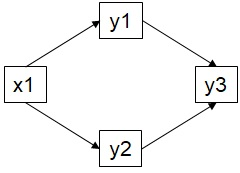2.2 GLM SEM In this SEM, there are two independence claims: 1. y3 | x1 (y1, y2) 2. y2 | y1 (x1) Considering the second independence claim, in a Gaussian world, the significance value is the same whether the test is conducted as y2 | y1 (x1) or y1 | y2 (x1). This is NOT true, however, when one or both of the variables are modeled using a generalized linear model (GLM) fit to a non-normal distribution. This is because the response is now transformed via the link function (see Section 2.2). This transformations means the P-value obtained by regressing y1 against y2 is NOT the same as the one obtained by regressing y2 against y1. The following example will show this is the case: # Generate fake data glmdat <- data.frame(x1 = runif(50), y1 = rpois(50, 10), y2 = rpois(50, 50), y3 = runif(50)) # Extract P-values summary(lm(y1 ~ y2 + x1, glmdat))$coefficients[2, 4]
##  0.6707592
summary(lm(y2 ~ y1 + x1, glmdat))$coefficients[2, 4] ##  0.6707592 # Repeat but model y1 and y2 and Poisson-distributed summary(glm(y1 ~ y2 + x1, "poisson", glmdat))$coefficients[2, 4]
##  0.6291832
summary(glm(y2 ~ y1 + x1, "poisson", glmdat))$coefficients[2, 4] ##  0.6163712 This effect is problematic because the d-sep tests are wholly dependent on the significance value. If the P-value is biased based on the direction of the test, then the goodness-of-fit of the model can be over- or underestimated. piecewiseSEM version 2.0 solves this by providing three options to the user. 1. One can specify the directionality of the test if, for instance, it makes greater biological sense to test y1 against y2 instead of the reverse. 2. One can remove that path from the basis set and instead specify it as a correlated error using %~~%. 3. One can conduct both tests and choose the most conservative (i.e., lowest) P-value. These options are returned by summary in the event the above scenario is identified in the SEM: # Generate fake data glmdat <- data.frame(x1 = runif(50), y1 = rpois(50, 10), y2 = rpois(50, 50), y3 = runif(50)) # Construct SEM glmsem <- psem( glm(y1 ~ x1, "poisson", glmdat), glm(y2 ~ x1, "poisson", glmdat), lm(y3 ~ y1 + y2, glmdat) ) summary(glmsem) ## Error: ## Non-linearities detected in the basis set where P-values are not symmetrical. ## This can bias the outcome of the tests of directed separation. ## ## Offending independence claims: ## y2 <- y1 *OR* y2 -> y1 ## ## Option 1: Specify directionality using argument 'direction = c()' in 'summary'. ## ## Option 2: Remove path from the basis set by specifying as a correlated error using '%~~%' in 'psem'. ## ## Option 3 (recommended): Use argument 'conserve = TRUE' in 'summary' to compute both tests, and return the most conservative P-value. In option 1, the directionality can be specified using direction = c() as an additional argument. summary(glmsem, direction = c("y1 <- y2"), .progressBar = F)$dTable
##   Independ.Claim Test.Type DF Crit.Value P.Value
## 1  y3 ~ x1 + ...      coef 46     1.1015  0.2764
## 2  y2 ~ y1 + ...      coef 47    -0.9263  0.3543

In option 2, the SEM can be updated to remove that test by specifying it as a correlated error (see Section 2.4).

summary(update(glmsem, y1 %~~% y2), .progressBar = F)
##
## Structural Equation Model of update(glmsem, y1 %~~% y2)
##
## Call:
##   y1 ~ x1
##   y2 ~ x1
##   y3 ~ y1 + y2
##   y1 ~~ y2
##
##     AIC      BIC
##  18.572   33.868
##
## ---
## Tests of directed separation:
##
##   Independ.Claim Test.Type DF Crit.Value P.Value
##    y3 ~ x1 + ...      coef 46     1.1015  0.2764
##
## Global goodness-of-fit:
##
##   Fisher's C = 2.572 with P-value = 0.276 and on 2 degrees of freedom
##
## ---
## Coefficients:
##
##   Response Predictor Estimate Std.Error DF Crit.Value P.Value Std.Estimate
##         y1        x1  -0.0493    0.1862 48    -0.2648  0.7912            -
##         y2        x1   0.0451    0.0812 48     0.5552  0.5787            -
##         y3        y1   0.0143    0.0173 47     0.8267  0.4126       0.1212
##         y3        y2  -0.0002    0.0074 47    -0.0209  0.9835      -0.0031
##       ~~y1      ~~y2  -0.1551         - 50    -1.0763  0.1437      -0.1551
##
##   Signif. codes:  0 '***' 0.001 '**' 0.01 '*' 0.05
##
## ---
## Individual R-squared:
##
##   Response     method R.squared
##         y1 nagelkerke      0.00
##         y2 nagelkerke      0.01
##         y3       none      0.01

Note that the claim no longer appears in the section for the tests of directed separation.

Finally, option 3 can be invoked by specifying conserve = T as an additional argument

summary(glmsem, conserve = T, .progressBar = F)$dTable ## Independ.Claim Test.Type DF Crit.Value P.Value ## 1 y3 ~ x1 + ... coef 46 1.1015 0.2764 ## 2 y2 ~ y1 + ... coef 47 -0.9263 0.3543 The user should be vigilant for these kinds of situations and ensure that both the specified paths AND the independence claims all make biological sense. In the case where the underlying assumptions of the d-sep tests can bias the goodness-of-fit statistic, piecewiseSEM should automatically alert the user. ### 2.4 Correlated errors Correlated errors reflect the situation where the relationship among the two variables is not presumed to be causal and unidirectional, but rather that both are being driven by some underlying driver and are therefore appear correlated. Such a relationship is denoted by using a double-headed arrow: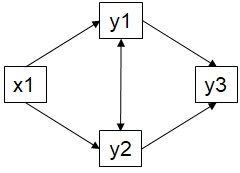2.4 Correlated error This behavior is specified in piecewiseSEM using the new operator %~~% in the psem function. We can fit the above SEM: cordat <- data.frame(x1 = runif(50), y1 = runif(50), y2 = runif(50), y3 = runif(50)) corsem <- psem( lm(y1 ~ x1, cordat), lm(y2 ~ x1, cordat), y1 %~~% y2, lm(y3 ~ y1 + y2, cordat) ) summary(corsem, .progressBar = F) ## ## Structural Equation Model of corsem ## ## Call: ## y1 ~ x1 ## y2 ~ x1 ## y1 ~~ y2 ## y3 ~ y1 + y2 ## ## AIC BIC ## 20.106 39.226 ## ## --- ## Tests of directed separation: ## ## Independ.Claim Test.Type DF Crit.Value P.Value ## y3 ~ x1 + ... coef 46 -0.0653 0.9482 ## ## Global goodness-of-fit: ## ## Fisher's C = 0.106 with P-value = 0.948 and on 2 degrees of freedom ## ## --- ## Coefficients: ## ## Response Predictor Estimate Std.Error DF Crit.Value P.Value Std.Estimate ## y1 x1 0.2283 0.1456 48 1.5675 0.1236 0.2207 ## y2 x1 -0.0526 0.1541 48 -0.3415 0.7342 -0.0492 ## ~~y1 ~~y2 0.0230 - 50 0.1580 0.4375 0.0230 ## y3 y1 -0.1702 0.1534 47 -1.1101 0.2726 -0.1576 ## y3 y2 0.1757 0.1484 47 1.1836 0.2425 0.1681 ## ## Signif. codes: 0 '***' 0.001 '**' 0.01 '*' 0.05 ## ## --- ## Individual R-squared: ## ## Response method R.squared ## y1 none 0.05 ## y2 none 0.00 ## y3 none 0.05 In the case where the correlated error occurs between two exogenous variables, it is simply the raw bivariate correlation whose P-value is determined using modifications to the function cor.test. In the event the correlated error includes an endogenous variable, it is the partial correlation that removes the effect of any covariates. In the above example, the correlated error removes the influence of x1 on both y1 and y2 before computing their correlation. cor(resid(lm(y1 ~ x1, cordat)), resid(lm(y2 ~ x1, cordat))) ##  0.02304697 cerror(y1 %~~% y2, corsem) ## Response Predictor Estimate Std.Error DF Crit.Value P.Value ## 1 ~~y1 ~~y2 0.02304697 NA 50 0.158044 0.4375496 ### 2.5 Nested models and AIC As noted, Shipley (2013) used the Fisher’s C statistic to construct an AIC score to facilitate model comparison and selection. This can be accomplished in the piecewiseSEM package with one important distinction. Let’s consider comparing the following models for the mediating role of y1: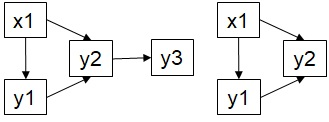2.5.1 AIC SEM One might think that the models could be coded like this, and then compared: AICdat <- data.frame(x1 = runif(50), y1 = runif(50), y2 = runif(50), y3 = runif(50)) sem1 <- psem( lm(y1 ~ x1, AICdat), lm(y2 ~ y1, AICdat), lm(y3 ~ y2, AICdat) ) sem2 <- psem( lm(y1 ~ x1, AICdat), lm(y2 ~ y1, AICdat) ) AIC(sem1, sem2) However, this does not account for the potential missing relationships with y3 to be in the model, which is critical as AIC incorporates Fisher’s C, which is determined by the d-sep tests. y3 must be present in the d-sep tests to make the comparison fair (i.e., the models must be nested).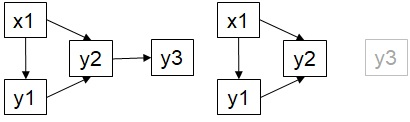2.5.2 AIC SEM (nested) To do so, we can use the following syntax: sem2new <- update(sem2, y3 ~ 1) AIC(sem1, sem2new) ## df AIC ## x 9 21.086 ## y 6 14.464 Now the comparison is fair and the model selection procedure is robust. Comparison of saturated models–ones that have no missing paths–and unsaturated ones using AIC is currently not possible, although we are looking into possible likelihood formulations in the absence of a C statistic (e.g., AIC = 0 + 2K). ## 3. Comparing Package Versions The new version 2.0 of piecewiseSEM replaces all of the old functions from version 1.x. This section will walk the user through the same worked example included in version 1.x from Shipley (2009), emphasizing the new syntax. ### 3.1 Introduction to Shipley (2009) Shipley included an example dataset in his 2009 paper ‘Confirmatory path analysis in a generalized multilevel context.’ The data are included in this version of the package and alternately hosted in Ecological Archives E090-028-S1 (DOI: 10.1890/08-1034.1). While not actual observations (the data are randomly generated), the hypotheses correspond to actual ecological phenomenon. Briefly, the data concern a forest survey where 5 individual trees of a particular species are followed at 20 sites every year beginning in 1970. For each individual, there is a measurement of: the latitude of the site (lat) the degree days until break (DD) the Julian date (date of year) of bud break (Date) the increase in stem diameter per tree (Growth) *a binary variable indicating whether the tree is alive (1) or dead (0) (Survival) Shipley notes that this model would be difficult to test using traditional SEM because it represents variation occurring at multiple levels (between sites, between individuals within sites, and between years within individuals within sites), and one response, survival, is not normally distributed. Shipley hypothesizes that the data adhere to the following hypothesized causal structure: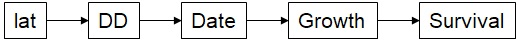3.1 Shipley SEM This example was included as the primary worked dataset in version 1.x of piecewiseSEM. ### 3.2 Comparing versions in evaluating the Shipley’s SEM In the previous version 1.x of the package, the model is constructed using a list and then supplied to various additional functions to measure fit, extract coefficients, and so on. Version 2.0 of the package uses the new function psem and uses summary to extract all that information at once. Next, we will walk through the analysis of the Shipley data using version 1.x and version 2.0. # Load required packages library(nlme) library(lme4) # Load Shipley data data(shipley) # Create list of structural equations shipley.list <- list( lme(DD ~ lat, random = ~ 1 | site / tree, na.action = na.omit, data = shipley), lme(Date ~ DD, random = ~ 1 | site / tree, na.action = na.omit, data = shipley), lme(Growth ~ Date, random = ~ 1 | site / tree, na.action = na.omit, data = shipley), glmer(Live ~ Growth + (1 | site) + (1 | tree), family = binomial(link = "logit"), data = shipley) ) Note the use of mixed effects models to account for the nested non-independence of replicates as well as the use of a generalized linear mixed effects model to account for the binomial distribution of survival. Next, we would have extracted the goodness-of-fit and path coefficients using the old code (deprecated). # (old.fit <- sem.fit(shipley.list, shipley, .progressBar = F)) # (old.coefs <- sem.coefs(shipley.list)) Now, we repeat the exercise using the new functions. as.psem converts the list to psem object, but you could also run the code using psem instead of list. shipley.psem <- as.psem(shipley.list) ### NOT RUN # shipley.psem <- psem( # # lme(DD ~ lat, random = ~ 1 | site / tree, na.action = na.omit, # data = shipley), # # lme(Date ~ DD, random = ~ 1 | site / tree, na.action = na.omit, # data = shipley), # # lme(Growth ~ Date, random = ~ 1 | site / tree, na.action = na.omit, # data = shipley), # # glmer(Live ~ Growth + (1 | site) + (1 | tree), # family = binomial(link = "logit"), data = shipley) # # ) Now we extract a summary of the object. (new.summary <- summary(shipley.psem, .progressBar = F)) ## boundary (singular) fit: see ?isSingular ## boundary (singular) fit: see ?isSingular ## ## Structural Equation Model of shipley.psem ## ## Call: ## DD ~ lat ## Date ~ DD ## Growth ~ Date ## Live ~ Growth ## ## AIC BIC ## 49.536 149.592 ## ## --- ## Tests of directed separation: ## ## Independ.Claim Test.Type DF Crit.Value P.Value ## Date ~ lat + ... coef 18 -0.0798 0.9373 ## Growth ~ lat + ... coef 18 -0.8929 0.3837 ## Live ~ lat + ... coef 1431 1.0280 0.3039 ## Growth ~ DD + ... coef 1329 -0.2967 0.7667 ## Live ~ DD + ... coef 1431 1.0046 0.3151 ## Live ~ Date + ... coef 1431 -1.5617 0.1184 ## ## Global goodness-of-fit: ## ## Fisher's C = 11.536 with P-value = 0.484 and on 12 degrees of freedom ## ## --- ## Coefficients: ## ## Response Predictor Estimate Std.Error DF Crit.Value P.Value Std.Estimate ## DD lat -0.8355 0.1194 18 -6.9960 0 -0.6877 *** ## Date DD -0.4976 0.0049 1330 -100.8757 0 -0.6281 *** ## Growth Date 0.3007 0.0266 1330 11.2917 0 0.3824 *** ## Live Growth 0.3479 0.0584 1431 5.9552 0 - *** ## ## Signif. codes: 0 '***' 0.001 '**' 0.01 '*' 0.05 ## ## --- ## Individual R-squared: ## ## Response method Marginal Conditional ## DD none 0.49 0.70 ## Date none 0.41 0.98 ## Growth none 0.11 0.84 ## Live delta 0.16 0.18 The output will look familiar to anyone who has run a regression in R. First, we have the call, which represents the structural questions. Next are the AIC and new BIC values, formerly accessible using sem.aic. The two produce identical values. # Old function # sem.aic(shipley.list, shipley, .progressBar = F)$AIC

# Extract from new summary object
new.summary$IC$AIC
##  49.536
### NOT RUN

# Alternately, one could call AIC() on the psem object
# AIC(shipley.psem)

Note that because the values are stored in the summary output, they are much quicker to access than having to recompute the d-sep tests using sem.aic.

Following the information criterion values, we have the tests of directed separation. As in the previous version, the independence claims are truncated to remove the conditioning variables (they can be shown using the argument conditional = TRUE).

The d-sep tests are, once again, identical between the two versions, but are faster to retrieve from summary than recomputing them from scratch.

# Old function
# sem.missing.paths(shipley.list, shipley, .progressBar = F)

# Extract from new summary object
new.summary$dTable ## Independ.Claim Test.Type DF Crit.Value P.Value ## 1 Date ~ lat + ... coef 18 -0.0798 0.9373 ## 2 Growth ~ lat + ... coef 18 -0.8929 0.3837 ## 3 Live ~ lat + ... coef 1431 1.0280 0.3039 ## 4 Growth ~ DD + ... coef 1329 -0.2967 0.7667 ## 5 Live ~ DD + ... coef 1431 1.0046 0.3151 ## 6 Live ~ Date + ... coef 1431 -1.5617 0.1184 ### NOT RUN # Alternately, one could call dSep() on the psem object # dSep(shipley.psem) After the d-sep table is the Fisher’s C statistic, and the results from the $$\chi^2$$ test. This information was formerly obtained from sem.fit. # Old function # sem.fit(shipley.list, shipley, .progressBar = F)$Fisher.C

# Extract from summary object
new.summary$Cstat ## Fisher.C df P.Value ## 1 11.536 12 0.484 As with all other functions, the values are exactly the same although the underlying functions have been rewritten to be more efficient. Next, we have the path coefficients and the standardized estimates. # Old function # sem.coefs(shipley.list, shipley) # sem.coefs(shipley.list, shipley, standardize = "scale") # Extract from new summary object new.summary$coefficients
##   Response Predictor Estimate Std.Error   DF Crit.Value P.Value Std.Estimate
## 1       DD       lat  -0.8355    0.1194   18    -6.9960       0      -0.6877
## 2     Date        DD  -0.4976    0.0049 1330  -100.8757       0      -0.6281
## 3   Growth      Date   0.3007    0.0266 1330    11.2917       0       0.3824
## 4     Live    Growth   0.3479    0.0584 1431     5.9552       0            -
##
## 1 ***
## 2 ***
## 3 ***
## 4 ***
# The new coefs() function is much faster too
coefs(shipley.psem)
##   Response Predictor Estimate Std.Error   DF Crit.Value P.Value Std.Estimate
## 1       DD       lat  -0.8355    0.1194   18    -6.9960       0      -0.6877
## 2     Date        DD  -0.4976    0.0049 1330  -100.8757       0      -0.6281
## 3   Growth      Date   0.3007    0.0266 1330    11.2917       0       0.3824
## 4     Live    Growth   0.3479    0.0584 1431     5.9552       0            -
##
## 1 ***
## 2 ***
## 3 ***
## 4 ***

Note two items: the standardized estimates and their standard errors are now reported by default at the end of the coefficients table (column Std.Estimate). the standardized estimate for the logistic regression is different, based on new calculations (see Section 2.2)

For the moment, version 2.0 only reports the scaled estimates (based on the standard deviations of the response and predictor). Future versions will include a range standardization.

Finally, summary reports the individual model R2 values. These were formerly obtained using sem.model.fits which was confusing. I have not included the old calculations in the package as the new rsquared function includes new calculations. However, this information is useful and therefore is now reported alongside the d-sep tests, Fisher’s C, and path coefficients.

The function partial.resid has been replaced with partialResid although the syntax is the same. The plotting output, however, has been eliminated.

partialResid extracts the partial effects of y ~ x in a multiple regression y ~ x + Z. For example:

# Generate data
dat <- data.frame(y = rnorm(100), x1 = rnorm(100), x2 = rnorm(100))

# Build model
model <- lm(y ~ x1 + x2, dat)

# Compute partial residuals of y ~ x1
yresid <- resid(lm(y ~ x2, dat))

xresid <- resid(lm(x1 ~ x2, dat))

# Use partialResid
presid <- partialResid(y ~ x1, model)

par(mfrow = c(1, 2))

plot(yresid, xresid)

plot(presid) # identical plot!

sem.lavaan has not yet been ported to version 2.0, and it may not, as there was some confusion how multi-level models were translated to the variance-covariance framework (hint: they weren’t, only the formulae were transferred).

sem.plot has also not yet been ported to version 2.0, since it was of limited utility. It may make an appearance in the future.